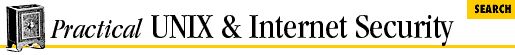home | O'Reilly's CD bookshelfs | FreeBSD | Linux | Cisco | Cisco ExamChapter 23Writing Secure SUID and Network Programs23.6 Tips on Generating Random Numbers

Random numbers play an important role in modern computer security. Many programs that use encryption need a good source of random numbers for producing session keys. For example, the PGP program uses random numbers for generating a random key which is used to encrypt the contents of electronic mail messages; the random key is then itself encrypted using the recipient's public key.

Random numbers have other uses in computer security as well. A variety of authentication protocols require that the computer create a random number, encrypt it, and send it to the user. The user must then decrypt the number, perform a mathematical operation on it, re-encrypt the number, and send it back to the computer.

A great deal is known about random numbers. Here are some general rules of thumb:

1. If a number is random, then each bit of that number should have an equal probability of being a 0 or a 1.

2. If a number is random, then after each 0 in that number there should be an equal proba- bility that the following bit is a 0 or a 1. Likewise, after each 1 there should be an equal probability that the following bit is a 0 or a 1.

3. If the number has a large number of bits, then roughly half of the number's bits should be 0s, and half of the bits should be 1s.

For security-related purposes, a further requirement for random numbers is unpredictability :

1. It should not be possible to predict the output of the random number generator given previous outputs or other knowledge about the computer generating the random numbers.

2. It should not be possible to determine the internal state of the random number generator.

3. It should not be possible to replicate the initial state of the random number generator, or to reseed the generator with the same initial value.

One of the best ways of generating a stream of random numbers is to make use of a random process, such as radioactive decay. Unfortunately, most UNIX computers are not equipped with Geiger counters. Thus, they need to use something else. Often, they use pseudorandom functions as random number generators.

A pseudorandom function is a function that yields a series of outputs which appears to be unpredictable. In practice, these functions maintain a large internal state from which the output is calculated. Each time a new number is generated, the internal state is changed. The function's initial state is referred to as its seed .

If you need a series of random numbers that is repeatable, you need a pseudorandom generator that takes a seed and keeps an internal state. If you need a non-reproducible series of random numbers, you should avoid pseudo- random generators. Thus, successfully picking random numbers in the UNIX environment depends on two things: picking the right random number generator, and then picking a different seed each time the program is run.23.5 Tips on Using Passwords23.7 UNIX Pseudo-Random Functions